# Excel IF OR function with formula examples

The tutorial shows how to write an IF OR statement in Excel to check for various "this OR that" conditions.

IF is one of the most popular Excel functions and very useful on its own. Combined with the logical functions such as AND, OR, and NOT, the IF function has even more value because it allows testing multiple conditions in desired combinations. In this tutorial, we will focus on using IF-and-OR formula in Excel.

## IF OR statement in Excel

To evaluate two or more conditions and return one result if any of the conditions is TRUE, and another result if all the conditions are FALSE, embed the OR function in the logical test of IF:

IF(OR(condition1, condition2,...), value_if_true, value_if_false)

In plain English, the formula's logic can be formulated as follows: If a cell is "this" OR "that", take one action, if not then do something else.

Here's is an example of the IF OR formula in the simplest form:

`=IF(OR(B2="delivered", B2="paid"), "Closed", "Open")`

What the formula says is this: If cell B2 contains "delivered" or "paid", mark the order as "Closed", otherwise "Open".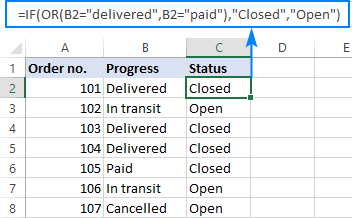In case you want to return nothing if the logical test evaluates to FALSE, include an empty string ("") in the last argument:

`=IF(OR(B2="delivered", B2="paid"), "Closed", "")`

The same formula can also be written in a more compact form using an array constant:

`=IF(OR(B2={"delivered","paid"}), "Closed", "")`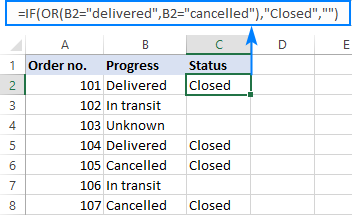In case the last argument is omitted, the formula will display FALSE when none of the conditions is met.

Note. Please pay attention that an IF OR formula in Excel does not differentiate between lowercase and uppercase characters because the OR function is case-insensitive. In our case, "delivered", "Delivered", and "DELIVERED", are all deemed the same word. If you'd like to distinguish text case, wrap each argument of the OR function into EXACT as shown in this example.

## Excel IF OR formula examples

Below you will find a few more examples of using Excel IF and OR functions together that will give you more ideas about what kind of logical tests you could run.

### Formula 1. IF with multiple OR conditions

There is no specific limit to the number of OR conditions embedded into an IF formula as long as it is in compliance with the general limitations of Excel:

• In Excel 2016 - 2007 formulas, up to 255 arguments are allowed, with a total length not exceeding 8,192 characters.
• In Excel 2003 and lower, you can use up to 30 arguments, and a total length shall not exceed 1,024 characters.

As an example, let's check columns A, B and C for blank cells, and return "Incomplete" if at least one of the 3 cells is blank. The task can be accomplished with the following IF OR function:

`=IF(OR(A2="",B2="", C2=""),"Incomplete","")`

And the result will look similar to this: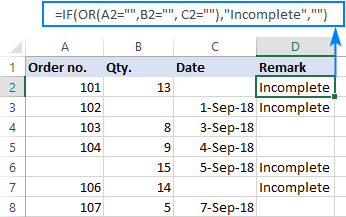### Formula 2. If a cell is this OR that, then calculate

Looking for a formula that can do something more complex than return a predefined text? Just nest another function or arithmetic equation in the value_if_true and/or value_if_false arguments of IF.

Say, you calculate the total amount for an order (Qty. multiplied by Unit price) and you want to apply the 10% discount if either of these conditions is met:

• in B2 is greater than or equal to 10, or
• Unit Price in C2 is greater than or equal to \$5.

So, you use the OR function to check both conditions, and if the result is TRUE, decrease the total amount by 10% (B2*C2*0.9), otherwise return the full price (B2*C2):

`=IF(OR(B2>=10, C2>=5), B2*C2*0.9, B2*C2)`

Additionally, you could use the below formula to explicitly indicate the discounted orders:

`=IF(OR(B2>=10, C2>=5),"Yes", "No")`

The screenshot below shows both formulas in action: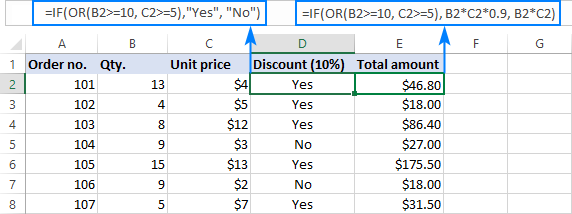### Formula 3. Case-sensitive IF OR formula

As already mentioned, the Excel OR function is case-insensitive by nature. However, your data might be case-sensitive and so you'd want to run case-sensitive OR tests. In this case, perform each individual logical test inside the EXACT function and nest those functions into the OR statement.

IF(OR(EXACT(cell,"condition1"), EXACT(cell,"condition2")), value_if_true, value_if_false)

In this example, let's find and mark the order IDs "AA-1" and "BB-1":

`=IF(OR(EXACT(A2, "AA-1"), EXACT(A2, "BB-1")), "x", "")`

As the result, only two orders IDs where the letters are all capital are marked with "x"; similar IDs such as "aa-1" or "Bb-1" are not flagged: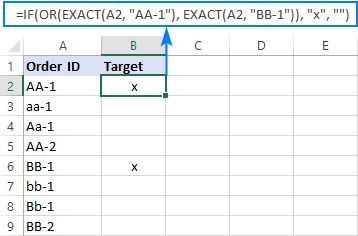### Formula 4. Nested IF OR statements in Excel

In situations when you want to test a few sets of OR criteria and return different values depending on the results of those tests, write an individual IF formula for each set of "this OR that" criteria, and nest those IF's into each other.

To demonstrate the concept, let's check the item names in column A and return "Fruit" for Apple or Orange and "Vegetable" for Tomato or Cucumber:

`=IF(OR(A2="apple", A2="orange"), "Fruit", IF(OR(A2="tomato", A2="cucumber"), "Vegetable", ""))`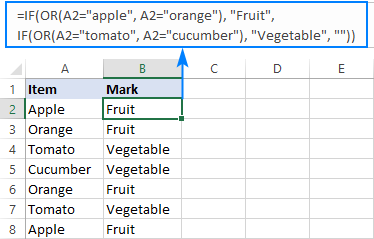### Formula 5. IF AND OR statement

To evaluate various combinations of different conditions, you can do AND as well as OR logical tests within a single formula.

As an example, we are going to flag rows where the item in column A is either Apple or Orange and the quantity in column B is greater than 10:

`=IF(AND(OR(A2="apple",A2="orange"), B2>10), "x", "")`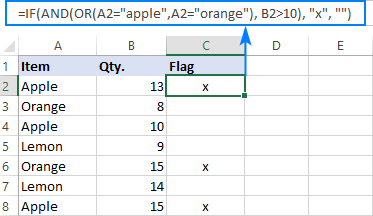That's how you use IF and OR functions together. To have a closer look at the formulas discussed in this short tutorial, you are welcome to download our sample Excel IF OR workbook. I thank you for reading and hope to see you on our blog next week!

## You may also be interested in

### 88 comments to "Excel IF OR function with formula examples"

1.NSN rao says:

i have customers data in excel how create customer wise statement a period of year or month

2.Leo says:

Hello there!

I could not get this formula to work. Could you help me identify where could be the error? It always gives a #VALUE! result.

=IF(OR(B:B={"Third Party & Terminal PIU Unit","Shaybah Projects Inspection Unit","Dist & Refined Product P/L PIU Unit","RT Refinery & Juaymah NGL Unit","RTR Clean Fuel Complex Unit","Riyadh Refinery Unit","Cross Country Pipeline PIU Unit","Master Gas System Proj Inspection Unit","Pipeline Upgrade & Crude Delivery Unit","WR Refining & NGL Projs Insp Unit","WR Pipelines & Terminal Unit","WR Bulk Plant & Dist Unit","Jazan Complex Projs Inspection Unit"}), "DPID", ""), IF(OR(B:B={"Maritime Yard Dev Project Inspection Sec","Ship Building Projects Inspection Unit","Off, Rigs Platform & Utls Proj Insp Unit","Maint & Support Vessels Proj Insp Unit","Special Kingdom Projects Inspection Unit","Community Projects Inspection Unit","Communication & Security Unit","Batch Plants & Civil Testing Unit"}), "MBIPID", ""), IF(OR(B:B={"Gas Compression Projs Inspection Sec","NA Gas Facilities","SA Gas Facilities","Fadhili Project Insp Unit","Hawiyah Increment Projs Inspn Unit","Haw/Una Gas Reservoir Storage PIU","North Gas Comp Plants Proj Insp Unit","Haradh&Hawiyah Comp P/L Proj Insp Unit","Satellite Gas Comp Plants Proj Insp Unit","South Gas Comp Plants Proj Insp Unit","Infrastructure & Support Proj Insp Unit","Jafurah Util, Sulfur & Intrcon Sys PIU","Jafurah Gas Processing Trains PIU","Jafurah Pipelines, IT & Site Dev PIU","Jafurah Infra & 3rd Party Coord PIU","Wasit-Jafurah NGL Fractionation PIU","Jafurah Pipelines, & Downstream Fac PIU","NGL Recovery & Fract' Unit","Utilities, Flare & Piperack Unit","Site Prep, ISF, SSF Unit","Inlet Storage & Compression Unit","Downstream Pipeline Unit","Gas Treat, Sulfur Rec' & Han' Fac Unit","Unconventional Resources Projs Insp Unit"}), "UGIPID", ""), IF(OR(B:B={"SA Oil MP Projs Inspection Unit","NA Oil MP Projs Inspection Unit","Gas MP Projs Inspection Unit","Marjan Offshore Gas Facilities Unit","Marjan GOSP-4 Unit","Marjan Offshore Oil Facilities Unit","Marjan Onshore Oil Facilities Unit","Zuluf Onshore Facilities Proj Insp Unit","Zuluf Offshore Facilities Proj Insp Unit","Infras, Pipeline & Comm Proj Insp Unit","North Ghawar Oil Facilities Unit","NA Oil Facilities","South Ghawar Oil Facilities Unit","Berri Increment Processing Fac Unit","Berri Onshore Facilities Unit","Fabyards ProJ Insp Unit","Installation Projects Insp Unit","Onshore Proj Insp Unit"}), "UOPID", "")

Many thanks.

•Alexander Trifuntov (Ablebits Team) says:

Hi!
Your formula is so big that it is impossible to understand it. It's not clear what you want to do. However, keep in mind that such a formula always returns an array of values.

=IF(A1:A10={"a","b","c"},TRUE)

See the result of this formula.

3.vivekr9741@gmail.com says:

“Gopal informed other students if you score 20 marks in end term exam OR 60 marks in total in
subject then you PASS otherwise FAIL.” write an excel command.

4.Tozy says:

I need help,
I have this scenario where Agent 1 has a ceiling of 500, Agent 2 has 250 and Agent 3 has 150.
If at anytime any of the agents pay goes above the ceiling, then 10% is calculated on the ceiling if the pay is below the ceiling then the 10% is calculated on that amount

How do i use IF statement to achieve this in Excel

•Alexander Trifuntov (Ablebits Team) says:

Hello!
I recommend reading this guide: Nested IF in Excel – formula with multiple conditions.
If I understand your task correctly, the following formula should work for you:

=IF(A1="Agent 1",IF(B1>500,500*10%,B1*10%),IF(A1="Agent 2",IF(B1>250,250*10%,B1*10%),IF(A1="Agent 3",IF(B1>150,150*10%,B1*10%))))

•Tozy says:

This works. Thank you

5.Jan says:

Hello,
Is there a way to combine two formulas below:
=IF(B63=TRUE; (G63)-(F63*1,21*D63); 0)
=IF(B63=TRUE; (G63)-(F63*1,21*D63); 0)

Tried this way, but it's not working:
=IF(B63=TRUE; (G63)-(F63*1,21*D63); 0); OR(=IF(B63=TRUE; (G63)-(F63*1,21*D63); 0))

•Jan says:

Sorry, mistake! I meant:

=IF(B63=TRUE; (G63)-(F63*1,21*D63); 0)
=IF(H63="Paid"; (G63)-(F63*1,21*D63); 0

Tried this way, but it's not working:
=IF(B63=TRUE; (G63)-(F63*1,21*D63); 0); OR(=IF(H63="Paid"; (G63)-(F63*1,21*D63); 0))

•Alexander Trifuntov (Ablebits Team) says:

Hi!
I think you have not read the article very carefully. There is an answer to your question.

=IF(OR(B63=TRUE;H63=”Paid”);(G63)-(F63*1,21*D63); 0)

•Jan says:

6.Dharmendar Rana says:

How To Extract Unique Values or Duplicate Names and sort (A-Z) Based On Criteria In Excel? Using index or match.

For Example:-

Sl No# Location Name score
1 Mumbai Rohit 93
2 Mumbai Sachin 93
3 Gujrat Suresh Raina 90
4 Ranchi M.S Dhoni 85
5 Ranchi Sorabh Tiwari 85

•Alexander Trifuntov (Ablebits Team) says:

Hello!
How to extract unique values using INDEX + MATCH functions, read this tutorial.

7.Office neophyte says:

Please check to see if the following is an error in the section "IF OR statement in Excel" where you state the lines below [in brackets like those enclosing this phrase to avoid confusion if I used double quotes]:

[ Here's is an example of the IF OR formula in the simplest form:

=IF(OR(B2="delivered", B2="paid"), "Closed", "Open")

What the formula says is this: If cell B2 contains "delivered" or "cancelled", mark the order as "Closed", otherwise "Open". ]

However, as I read the formula, it indicates that if cell B2 contains "delivered" or "paid" (not "cancelled") then the order will be marked as "Closed". If you look at the screen shot, the row containing "Cancelled" shows a Status of "Open", not "Closed" as your explanation states it will. Please clarify for your readers.

•Svetlana Cheusheva says:

Hello!

Of course, it is "paid", not "cancelled". Thank you for pointing that out, fixed!

8.Dawn R. Landron says:

I am trying to say that if One Cell = this amount add / subtract a Certain amount.

Can this be done??

EX: =IF(D6/7=E6,G6) OR (D6/7=E6,H6) OR (D6/7=E6,I6) OR (D6/7=E6,J6) OR (D6/7=E6,K6)

Thx...Dawn

•Alexander Trifuntov (Ablebits Team) says:

Hi!
Sorry, I cannot understand your formula

9.SAH VERMA says:

=IF(AND(A2="VISHAL", B2="HP", C2=610), "6", "10"), IF(AND(A2="VISHAL", B2="HP", C2=2310), "15", "20")

WILL THIS WORK?????????
I NEED TO ENTER MULTIPLE RESULT IN A SINGLE CELL, FROM DIFFERENT CONDITIONS.

•Alexander Trifuntov (Ablebits Team) says:

Hello!
Your conditions contradict each other. For example, if A2 = ”VISHAL”, B2 = ”HP”, C2 = 900 then the first condition will return 10, and the second - 20.

10.skye says:

This isn't working. What am I doing incorrectly?

=IF((OR(E2=Daily, E2=Weekly)), Next Shift, ENTER DATE)

Column E indicates if a project is due daily or weekly. Column F would ideally calculate today+1 for daily or today+8 days for weekly.

•Alexander Trifuntov (Ablebits Team) says:

Hi,
You must enclose text values in quotation marks, such as "Weekly".
What is "Next Shift, ENTER DATE"?
Based on your description, it is hard to completely understand your task. However, I’ll try to guess and offer you the following formula:

=IF(E2="Daily",TODAY()+1,IF(E2="Weekly",TODAY()+8,""))

Hope this is what you need.

11.Joyee says:

I got it! thank you. :)

12.Joyee says:

Hi.. need help.
i have date today and start date, to calculate the case age but another column is the status of the case, close or open.. so the logic will be.. calculate the case age if the case is still open..

•Joyee says:

I got this formula: =IF(OR(C2="Closed","--"),(SUM(A2-B2)))
but..
it's working but the other way around. it calculates the age if the case is marked as "Closed".

•Alexander Trifuntov (Ablebits Team) says:

Hello!
In the condition of the IF function, write down the check that the case is open. If the condition is met, calculate the age using the DATEDIF function.
Hope you’ll find this information helpful.

13.Nicole says:

Hi, I would like to know a formula to show if something if greater than or less than a number to show a figure for example

11 years service - if the years service is more than 10 to show 2, if it is less than 10 but more than 5 to show 1 and if it is less than 5 to show 0.

hope this makes sense.

TIA

•Alexander Trifuntov (Ablebits Team) says:

Hello!
I hope you have studied the recommendations in the tutorial above. It contains answers to your question.

=IF(A1>10,2,IF(A1>5,1,0))

•karim abbas says:

there are some proble with me in excell example
=if(a1<10,100,"enough",if(d1=0,"niu"))

14.Garnet mulita says:

Please I need your help how can I come up with the formula for this
45000 =0%
5000=15%
Next 2950000=30%
Excess 3000000=35%

•Alexander Trifuntov (Ablebits Team) says:

Hello!

15.Aira says:

I need some help in constructing the formula to this:
I need to derive a result(column title) if ALW(column title) is 1.56 and up its Oversize, if ALW is 1.20-1.55 its Goodsize, if ALW is 1.10-1.19 its Undersize, if ALW is 1.0-1.09 its Offsize, and if ALW is below 1.0 its Runts

•Alexander Trifuntov (Ablebits Team) says:

Hello!
What is column title? In Excel and other spreadsheet applications, the column header is the colored row of letters used to identify each columnwithin the sheet, or workbook. Column title is a letter.

=IF(A1>=1.56,"Oversize", IF(A1>=1.2,"Goodsize", IF(A1>=1.1,"Undersize", IF(A1>=1,"Offsize", "Runts" ))))

16.AJITHA says:

i need a formula like ( date of joinin - current date less than 365 days then the answer should be 0

17.Joanne says:

Hi,
I am trying to do the following if statements with the last if statement to add on an additional 1 week if P13 = "U" but I can't get this to work. Any help would be welcomed.
=IF(AND(O131,O133,O135),4,IF(AND(P13="U",2),TRUE)))))

•Joanne says:

Hi,
I am trying to do the following if statements with the last if statement to add on an additional 1 week if P13 = "U" but I can't get this to work. Any help would be welcomed.
=IF(AND(O131,O133,O135),4,IF(AND(P13="U",2),TRUE))))).
Thanks so much.

•Alexander Trifuntov (Ablebits Team) says:

Hello Joanne!
Please describe your problem in more detail. Include an example of the source data and the result you want to get. It’ll help me understand your request better and find a solution for you. Thank you.

18.SARAVANAN says:

IF(A1="DELIVERY",THEN C1(CELL NO)*.020%,IF NO C1*.004% I NEED CORRECT FORMULA

19.Michael Albuquerque says:

How do I write the formula for... If either Cell A1 or Cell D1 contains a term, say "ENGLISH", then the consequent grade of ENGLISH from the C1 or F1 should be filled in cell G1.
Is it possible?

•Alexander Trifuntov (Ablebits Team) says:

Hello Michael!

=IF(A1="ENGLISH",C1, IF(D1="ENGLISH",F1,""))

20.A says:

=IF(E45="PA1",0.85,IF(E45="PA2",0.95,IF(E45="CB1",0.99,"")))

21.BRIAN says:

I NEED A FORMULA FOR CELL F45
IF CELL E45 = "PA1" THEN CELL F45= .85 AND IF CELL E45 = PA2 THEN CELL F45 READS .95 AND IF CELL = E45 - CB1 THEN F45 = .99

THANKS

22.Vickie says:

Trying to write a formula that picks out the word grapefruit from D14 or the word recorder from D14 and gives a zero.. if those words aren't found then F14-E14. The formula works for just Grapefruit but when I add in the Or and Recorder it doesn't. What am I writing wrong? It's telling me to many arguments.
=If(Or(Is number(Search("Grapefruit"'D14,(Is number(Search("Recorder",D14),0,F14-E14))

23.Sam V says:

Can I not make cell to cell comparison with if/or? Here is the formula I am using.
=IF(OR(D3<E3,D3=E3,"Good", "Review"))

•Sam V says:

Here is the formula I used after reviewing the responses to other questions on this forum. New formula works.
=IF(D3<=E3, "Good", "Review")

•Adrian says:

Hi Sam,
Your original formula would work as well. You just had to move the other bracket to close off the or( function.
=IF(OR(D3<E3,D3=E3),"Good", "Review")

24.Prakash Kulkarni says:

Can you help me on the error in this formula. =IF(ISNUMBER(\$AH15),ANDIF(\$AH15>150,(" High Random Blood Sugar "&\$AH15&" Mg.%. ", "")&" "&IF(\$AH15>150,"Urine Sugar "&\$AI15&". ", ""),("")
AH15 is Number or Text "ND" i.e. Not Done.
Thank you.

•Adrian says:

=IF(OR(ISNUMBER(\$AH15),\$AH15="ND"),IF(\$AH15>150,"High Random Blood Sugar"&\$AH15&"Mg.%.","")&" "&IF(\$AH15>150,"Urine Sugar"&" "&\$AI15&".",""),"")
The above formula seems to work for me.

25.Sondra Riggs says:

I have a price range for warranty coverage. I need to see when sales either sold the item over or under the range for a warranty package. For example:
Min Product \$ Range Max Product \$ Range Product \$ Sold
1000 1499.99 269.00
300 599.99 1049.00
1000 1499.99 578.00
600 799.99 1456.00

I need a formula that tells me if the product sold for \$269.00 was "oversold" or "undersold" contract range? I tried =if(or(c1=B2,"oversold"))
It doesn't work. What am I doing wrong?

•Adrian says:

A = Min / B = Max / C = Sold
=IF(C1B1,"OVERSOLD","")

•Adrian says:

Something is wrong with the formula not being posted properly.
=IF(C1B1,"OVERSOLD","")
It should be:
=IF(C1 less than A1,"UNDERSOLD",IF(C1 greater than B1,"OVERSOLD","")

26.Fatih says:

Hello,
I want to write a formula to write C1 as:
1 if A1>10 or B1>20
2 if 7<A1<10 or 10<B1<20
3 if 4<A1<7 or 5<B1<10
4 if 1<A1<4 or 1<B1<5
5 if A1<1 or B1<1

27.carter harden says:

I have student totals,I want to apply comments, 400 and above should have good performance, 300-400 should have fair performance, below 300 should have poor performance,the cell for total is I

28.Tina Owens says:

+1 when the value is >=5,
+2 when the value is >=10,
+3 when the value is >=15,
+4 when the value is >=20,
+5 when the value is >=25
The formula I am currently using is:
=IF(F7>=5,H7+1,IF(F7>=10,H7+2,IF(F7>=15,H7+3,IF(F7>=20,H7+4,H7))))
This formula is working for the +1 when the value is >=5, but when the value is >=10, it is still adding +1.

•Natalia Sharashova (Ablebits Team) says:

Hello Tina,

You see, your first condition fits to all other conditions as well - the value is greater than 5. You need to limit each condition and check, for example, if the number is not only greater than or equal to 5 but also less than 10.
=IF(AND(F7>=5,F7<10), H7+1, IF(AND(F7>=10,F7<15), H7+2, IF(AND(F7>=15,F7<20), H7+3, IF(F7>=20,H7+4, H7))))

You will find the info about the IF function in Google Sheets in this post.

29.Sue M says:

I have 2 columns, work email(D2) & personal email(E2). I am trying to create a formula in a new field (preferred email) that says if D2 is blank use E2 (if there is a value) or if E2 is blank use D2 or leave blank. Is this possible?

•Aksana Pachkouskaya (Ablebits Team) says:

Hi,
If I get it right, your task is as follows: if a cell in Column D contains an email address, a formula is to bring it; if not, it should bring an email address from a cell in Column E; if both cells are empty, the formula has to bring nothing. I hope you do not mind lengthy formulas:

=IFS(OR(AND(N(ISBLANK(D2))=0, N(ISBLANK(E2))=0), AND(N(ISBLANK(D2))=0, N(ISBLANK(E2))=1)), D2, AND(N(ISBLANK(D2))=1, N(ISBLANK(E2))=0), E2, AND(N(ISBLANK(D2))=1, N(ISBLANK(E2))=1), "")

•Svetlana Cheusheva says:

Hi Sue,

If you love compact formulas, use this one :)

=IF(D2<>"", D2, IF(E2<>"", E2, ""))

•Adrian says:

That's awesome.

30.Björne says:

In column C I enter one of 7 names.
Depending on the name I want different results in column N
So
If the name is
1 - Andy Black the result should be 400
2 - Mr Jet, Nina Sven or Mike Young the result should be 600
3 - Dr Joe, Miss Adams or Neil Foe the result should be 800
4 - Ms Hard the result should be 1000
5 - Mr Woo the result should be 1200

Which formula do I use to solve this?

•Aksana Pachkouskaya (Ablebits Team) says:

Hi Björne,
The following formula suggests itself:

=IFS(C2="Andy Black", 400, OR(C2="Mr Jet", C2="Nina Sven", C2="Mike Young"), 600, OR(C2="Dr Joe", C2="Miss Adams", C2="Neil Foe"), 800, C2="Ms Hard", 1000, C2="Mr Woo", 1200)

31.aimo says:

Formula 2. If a cell is this OR that, then calculate
=IF(OR(D3>0,D390,D3180,D3270,D30,D390,D3180,D3270,D3<360),W3] are not coming
- need to know what to change to make it work .. than you

32.jwalker says:

I am looking for the correct formula to use to return the greatest of two values. For example, if Q3 (5.89) is greater than R3 (7.452), I want S3 to show R3 value (7.452). If Q17 (28.86) is greater than R17 (3.105), I want S17 to show Q17 value (28.86).

•Aksana Pachkouskaya (Ablebits Team) says:

Hi Jwalker,
I hope that your task may be expressed in the following way: if the value in Cell R3 is less than the value in Cell Q3, the value from Cell Q3 is needed; if the value in Cell R3 is more than the value in Cell Q3, the value from Cell R3 is needed. If so, here is the formula you could apply:

=IF(Q3 < R3, R3, Q3)

33.MuiMui says:

I need to validate customer order to ensure it is not less than minimum order value(MOV) and not less than minimum order qty. We validated order value on one column, filter out the order lines with order value lower than MOV and then validated order qty on another column. Is there shortcut to have all the validation performed under single column with a sophisticated nested if function?

34.farzaneh says:

hi everyone,
how can i formulate this one?
if >=6 : full assistance
if =4 or 5 : half assistance
if <= 3 : no assistance

•Aksana Pachkouskaya (Ablebits Team) says:

Hi Farzaneh,
I hope the following formula will do the job:

=IFS(D2<=3, "no assistance", OR(D2=4,D2=5), "half assistance", D2>=6, "full assistance")

35.Marty says:

So how would I do this? If cell A1 is equal to 10, I want to multiply B1 by ten, but if A1 is equal to 25 I want to multiply B1 by four, but if A1 is equal to 50 I want to multiply B1 by 2.

36.Vikash says:

Hi all
I want to differentiate the cell values into the crores, Lakh, Thousand, Hundred, Tens, Units
Example : -
123456789
12 Crores 24 Lakh 56 Thousand 7 Hundred 89
So how will i do can anyone here who can help me

37.Mark Whiteley says:

Hi, Hoping someone can help.
I'm trying to write a formula using the IF, AND, or OR function but can't get the formula correct. It should be simple, really.
The conditions are;
If the SUM of Cells E4:G4 = between 10 and 15, then Cell G14 = 25
If the SUM of Cells E4:G4 is greater than or equal to 15, then Cell G14 = 50

Then there's one other result that I'm trying to achieve (in a separate cell but a similar formula)
If the SUM of Cells E4:P4 = between 400 and 600, then Cell P15 = 10
If the SUM of Cells E4:P4 is greater than or equal to 600, then Cell P15 = 20
I can't work out what I'm doing wrong, I wont paste what formulas i currently have to avoid causing a case of mass confusion.

•Adrian says:

=IF(AND(SUM(E4:G4>10),SUM(E4:G4=15,50,"")))

=IF(AND(SUM(E4:P4>400),SUM(E4:P4=600,20,"")))

•Adrian says:

=IF(AND(SUM(E4:G4)>10,SUM(E4:G4)15,50,""))

=IF(AND(SUM(E4:P4)>400,SUM(E4:P4)600,25,""))

Completely butchered the original answer. This one should work.

38.Laura says:

I am trying to evaluate if the first date is a weekend or the time is after 5pm.
Each works on its own but is not working when combines with the OR
I am getting #NAME?
=IF(OR(WEEKEND(E2,2)>5,K19>TIME(17,0,0)),"OT", "REG")

Any Ideas

•Laura says:

Apparently I just needed to retype and press the keyboard harder:) it worked the 50th time I typed it I don't know why as it looks exactly the same. But for now I will move on.

39.Peter says:

If F34 value = "Dealer", then used values Column K OR
If F34 value = "Trade", then use values Column M OR
If F34 value = "End User", then use values Column

40.Brett says:

If I want to reference three cells, what's the formula? (i.e =IF(F113-"x",(J126)),=IF(G113-"x",(K126)),=IF(H113-"x",(K126))

H126 want to be the value of one of three cells depending the selection of another value in three cells

41.babarbuttgrt says:

Hi everyone

I need little help to construct formula from below pseudo code.

If{
customer = private AND account_status = active AND account_open_date >23-June-2006
THAN
If {
risk = high
THAN
Last Review date = 1st review date + 6 Months
}
Else If{
risk = medium
THAN
Last Review date = 1st review date + 12 Months
}
Else If{
risk = low
THAN
Last Review date = 1st review date + 12 Months
}
Else {
last review date = 24-June-2006
}
}
If {
customer = govt AND account_open_date 23-June-2006
THAN
If {
risk = high
THAN
Last Review date = 1st review date + 6 Months
}
Else If {
risk = medium
THAN
Last Review date = 1st review date + 12 Months
}
Else If{
risk = low
THAN
Last Review date = 1st review date + 12 Months
}
}

42.Rick says:

I need the response in column D , labeled "link", to substitute the number of the column with the actual entry in that column of the row. The desired results, column E, are in the "want" column. Any thoughts?

J18.9 A41.9 1 J18.9
R41.82 E86.0 E43 2 E86.0
G20 R26.89 G30.9 1,3 G20, G30.9

43.Victor says:

Hello,

Thanks for your teach, but i think that is better to use brackets, especially if B2 can have several values

=IF(OR(B2={"delivered";"paid"});"Closed"; "")

=IF(OR(B2="delivered", B2="paid"), "Closed", "Open")

Regards

•Svetlana Cheusheva says:

Hi Victor,

I agree, this makes the formula more compact. Thanks for the tip!

•vinay says:

=IF(OR(B2={"delivered";"paid"}),"Closed","") correct

44.girineo1 says:

Hi. Can you help me. i have the following. Column Q is a sum of hours for operations. Column Y is my set hour reset. Y2 Starts at 120 hours and ends at Y23 at 2640 hours. increments are in 120 hours.
In Columm Q i have the following formula =Sum(K3+Q2). on R3 i want to add a formula to do the following.

If cell Q3 is 120240 then subtract Q3-\$Y\$2, or if Q3 is 241360 then Subtract Q3-\$Y\$3, or if Q3 is 361480 then subtract Q3-\$Y\$4, if false then add K3+Q2

Whats the best way for me to write it.

i manage to write a formula but it turns the cell in Column R when ever the statement is true "true".

45.Shobi Imran says:

Very good article, thanks for sharing, Keep up the good work!

46.Craig Gordon says:

Hi there

•Marty says:
•Marty says: